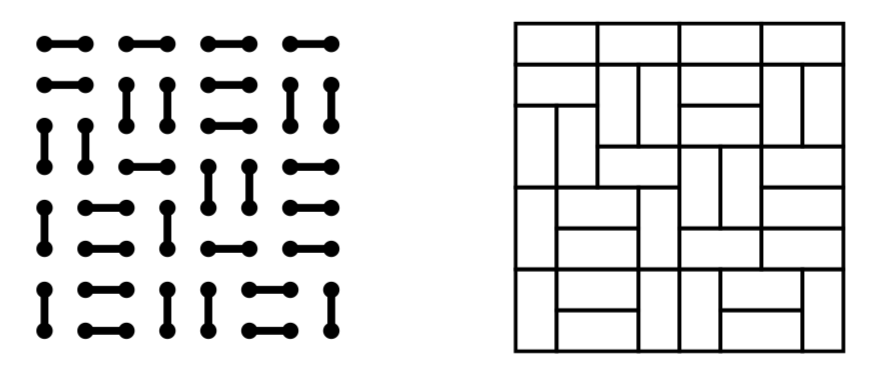# What is the link between the Domino Tilings and the Ising Model?

Is there a link between the theory of Domino Tilings and the Ising Model? In the global qualitative sense that physicists use, the answer is "yes". The connections could go like this:

• The dimer model is the limit of the ising model (Onsager?)
• The theory of domino tiling is dual to the theory of dimer tilings. (Kenyon?)

However, when I put the two connections together, I wasn't able to say this Ising model configuration maps to this Domino Tiling. And I suspect, they are slightly different objects. First here's the section from Baxter's book on Exactly Solved Models giving us hope:He tells us, the Ising Model "freezes" into a Dimer problem, which is dual to a domino tiling problem.

From Fisher's original 1966 paper we find, the square Ising model maps to dimers model on "decorated" square lattice:The theories of exactly solved statistical mechanics and of integrable systems are not necessarily related. It's just that if I read one, then I inadvertently start reading the other. Here's a discussion of Korepin and Izergin.So now, there is also a link between 6-vertex model and domino tilings. Does that also have fine print?

For all of the above questions, I cited papers from the 1960's and 1980's. I wonder what the modern perspective of integrable theories are.

I seem to have asked a question like this already. And there will be more clarifications yet to come.

• Do you have a particular lattice (and boundary conditions) in mind for the Ising model"? – Jules Lamers Nov 11 '17 at 20:24
• @JulesLamers so I am asking for a relationship between square-lattice ising model, square-lattice dimer model and square-lattice 6-vertex and 8-vertex models. I'm concluding that these are different models, maybe different "Hamiltonians" on the vertices (or edges , or faces) of the square lattice. – john mangual Nov 11 '17 at 20:48
• My name is Zinn-Justin, not Izergin! – Paul Zinn-Justin Jan 25 '18 at 11:46

For a more modern treatment of the links between dimers and Ising, and exactly where the small print is, I would advise you to read the habilitation memoir of Béatrice de Tilière from 2013, available at

http://perso.math.u-pem.fr/de_tiliere.beatrice/HDR/memoire.pdf

(and of course the references therein). But I would say that already the dimer model on the Fisher lattice gives the right idea that there is a deep connection between the two models.

• Oh yes. She's an expert! And Cédric – john mangual Nov 11 '17 at 20:16

Since others have already commented on the link with domino tilings, let me point out a relation between the eight-vertex model and an Ising-type model on the square lattice, cf the comments.

These Ising-type models are a bit unusual: they feature both two- and four-point interactions. [The latter are like the interactions of an interaction-round-a-face (IRF) model on the dual lattice.] The weights can be made to match, and the relation is 2-to-1 at the level of configurations, so the partition functions match up to a factor of two. See e.g. eight-vertex model at Wikipedia and the references there.

(As you know I explained the relation between the six-vertex model and domino tilings of the Aztec diamond, cf the last figure in the OP, in my answer to your question that you mention.)

The two problems of the enumeration of domino tilings and the calculation of the ground state of the Ising model are both problems that can be solved by finding perfect matchings of a planar graph, but the graphs are different. A domino tiling is a perfect matching of a square lattice, see this figure:The partition function of the ground state of the Ising model is given by the minimum-weight perfect matching for a decorated version of the square lattice, as in the figure shown in the OP.

For a modern treatment of the correspondence between Ising models and dimer models, see On Ising and dimer models in two and three dimensions (2003).